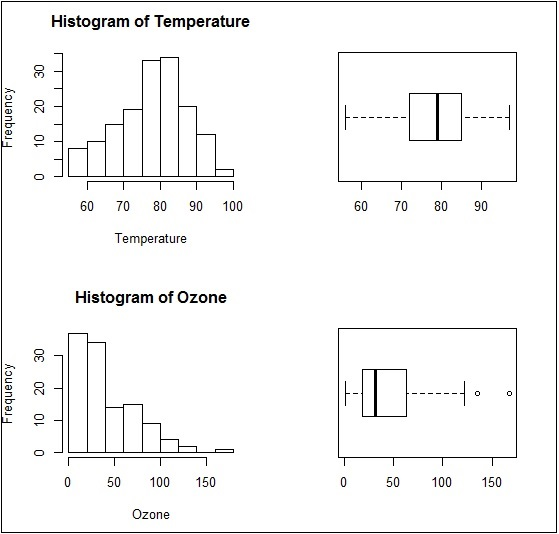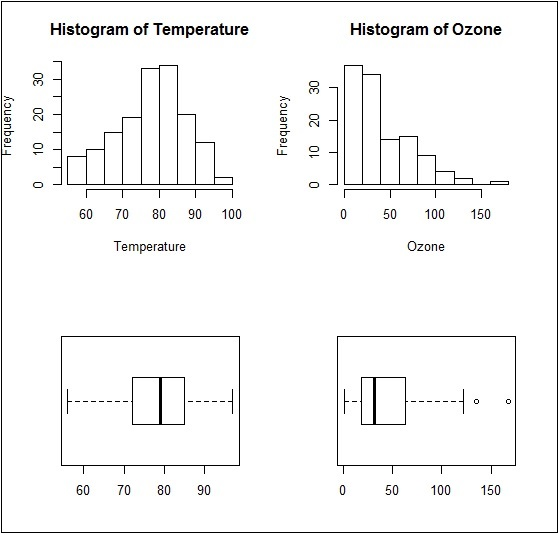# ggplot2 - Multi Panel Plots

Multi panel plots mean plot creation of multiple graphs together in a single plot. We will use par() function to put multiple graphs in a single plot by passing graphical parameters mfrow and mfcol.

Here we will use “AirQuality” dataset to implement multi panel plots. Let us understand the dataset first to have a look on creation of multi panel plots. This dataset includes Contains the responses of a gas multi-sensor device deployed on the field in an Italian city. Hourly responses averages are recorded along with gas concentrations references from a certified analyzer.

## Insight of par() function

Understand the par() function to create a dimension of required multi panel plots.

```> par(mfrow=c(1,2))
> # set the plotting area into a 1*2 array
```

This creates a blank plot with dimension of 1*2.Now create the bar plot and pie chart of the mentioned dataset using following command. This same phenomenon can be achieved with the graphical parameter mfcol.

## Creating Multi Panel Plots

The only difference between the two is that, mfrow fills in the subplot region row wise while mfcol fills it column wise.

```> Temperature <- airquality\$Temp
> Ozone <- airquality\$Ozone
> par(mfrow=c(2,2))
> hist(Temperature)
> boxplot(Temperature, horizontal=TRUE)
> hist(Ozone)
> boxplot(Ozone, horizontal=TRUE)
```The boxplots and barplots are created in single window basically creating a multi panel plots.

Same plot with a change of dimensions in par function would look as follows −

```par(mfcol = c(2, 2))
```